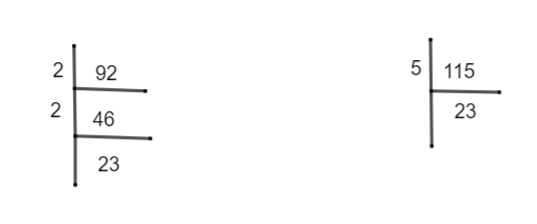Courses
Courses for Kids
Free study material
Free LIVE classes
MoreLIVE
Join Vedantu’s FREE Mastercalss

# The ratio of 92:115 in its simplest form is(a).23:25(b).18:25(c).3:5(d).4:5Verified
359.7k+ views
Hint:Take the common factors of 92 and 115 by prime factorization method. Cancel out the same terms in both the numbers. And you will get their simplified ratio.

A ratio is the quantitative relation between 2 amounts showing the number of times one value contains or is contained within the other i.e. a ratio indicates how many times one number contains another.
For example there are 4 boxes of 3 that are shaded and 1 is not shaded so their ratio 3 shaded box to 1 non-shaded box is 3:1, it can be also used the word “to” = 3 to 1.
It can be also written as a fraction$\dfrac{3}{1}$.
Given is the ratio 92:115, we need to simplify it.
Taking 92 let us find the common factors by using the prime factorization method.Similarly, let us find the common factors of 115.
\begin{align} & \therefore 92=2\times 2\times 23 \\ & 115=5\times 23 \\ \end{align}
We said that 92:115 can also be written in fraction $\dfrac{92}{115}$.
$\therefore \dfrac{92}{115}=\dfrac{2\times 2\times 23}{5\times 23}$
Cancel 23 from the numerator and the denominator.
$\therefore \dfrac{92}{115}=\dfrac{4}{5}$
$\therefore$ The simplified ratio of 92:115 is 4:5.
So, option (d) is correct.
Note:
We can always simplify or expand it.
4:5 ratio is similar to$4\times 2:5\times 2=8:10$.
8:10 ratio is similar to$8\times 3:10\times 3=24:30$.
If we are multiplying 23 on 4:5 $\Rightarrow 4\times 23:5\times 23$ we get 92:115.
Last updated date: 16th Sep 2023
Total views: 359.7k
Views today: 8.59k